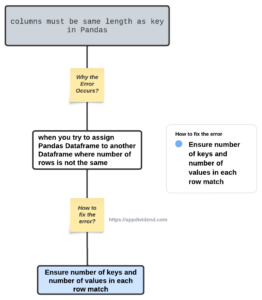# How to Fix ValueError: columns must be same length as key in PandasDiagram

To fix the ValueError: columns must be same length as key error, “ensure the number of keys and the number of values in each row match and that each key corresponds to a unique value.”

ValueError: columns must be same length as key error occurs when you “try to assign Pandas Dataframe to another Dataframe where the number of rows is not the same.”

## Common reasons for the error

1. When you attempt to assign a list-like object (For example, lists, tuples, sets, numpy arrays, and Pandas Series) to a list of DataFrame columns as new arrays, but the number of columns doesn’t match the second (or last) dimension (found using `np.shape`) of the list-like object.
2. When you attempt to assign a DataFrame to a list (or pandas Series or numpy array or pandas Index) of columns, the respective numbers of columns don’t match.
3. When you attempt to replace the values of an existing column with a DataFrame (or a list-like object) whose number of columns doesn’t match the number of columns it’s replacing.

## Reproduce the error

``````import pandas as pd

list1 = [11, 21, 19]
list2 = [[46, 51, 61], [71, 81, 91]]

df1 = pd.DataFrame(list1, columns=['column1'])
df2 = pd.DataFrame(list2, columns=['column2', 'column3', 'column4'])

df1[['a']] = df2``````

Output## How to fix it?

``````import pandas as pd

list1 = [11, 21, 19]
list2 = [[46, 51, 61], [71, 81, 91]]

df1 = pd.DataFrame(list1, columns=['column1'])

# Increase the number of rows in df1 to match the number of columns in df2
df1 = pd.concat([df1] * len(list2), ignore_index=True)

df2 = pd.DataFrame(list2, columns=['column2','column3','column4'])

df1[['column2', 'column3', 'column4']] = df2

print(df1)``````

Output

``````   column1  column2  column3  column4
0    11      46.0     51.0     61.0
1    21      71.0     81.0     91.0
2    19      NaN      NaN      NaN
3    11      NaN      NaN      NaN
4    21      NaN      NaN      NaN
5    19      NaN      NaN      NaN``````

That’s it!

This site uses Akismet to reduce spam. Learn how your comment data is processed.